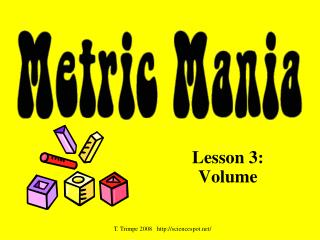# Lesson 3: Volume - PowerPoint PPT PresentationDownload PresentationLesson 3: Volume

Lesson 3: VolumeDownload Presentation## Lesson 3: Volume

- - - - - - - - - - - - - - - - - - - - - - - - - - - E N D - - - - - - - - - - - - - - - - - - - - - - - - - - -
##### Presentation Transcript

1. Lesson 3: Volume T. Trimpe 2008 http://sciencespot.net/

2. 1 fl oz = 29.573 ml 1 12-oz can of soda would equal approximately 355 ml. 1 quart = 0.946 liters 1 gallon = 3.79 liters It would take approximately 3 ¾ 1-liter bottles to equal a gallon. English vs. Metric Units Which is larger? A. 1 liter or 1 gallon B. 1 liter or 1 quart C. 1 milliliter or 1 fluid ounce

3. kL Metric Units cL mL L Volume is the amount of space an object takes up. The base unit of volume in the metric system in the liter and is represented by L or l. Standard: 1 liter is equal to one cubic decimeter Metric Units 1 liter (L) = 1000 milliliters (mL) 1 milliliter (mL) = 1 cm3 (or cc) = 1 gram* Which is larger? Click the image to watch a short video about volume. A. 1 liter or 1500 milliliters B. 200 milliliters or 1.2 liters C. 12 cm3 or 1.2 milliliters* * When referring to waterLiter Image: http://www.dmturner.org/Teacher/Pictures/liter.gif

4. What causes the meniscus? A concave meniscus occurs when the molecules of the liquid attract those of the container. The glass attracts the water on the sides. Measuring Volume We will be using graduated cylinders to find the volume of liquids and other objects. Read the measurement based on the bottom of the meniscus or curve. When using a real cylinder, make sure you are eye-level with the level of the water. What is the volume of water in the cylinder? _____mL Top Image: http://www.tea.state.tx.us/student.assessment/resources/online/2006/grade8/science/images/20graphicaa.gifBottom Image: http://morrisonlabs.com/meniscus.htm

5. Measuring Liquid Volume What is the volume of water in each cylinder? Images created at http://www.standards.dfes.gov.uk/primaryframework/downloads/SWF/measuring_cylinder.swf A B C Pay attention to the scales for each cylinder.

6. 9 cm 8 cm 10 cm We can measure the volume of irregular object using water displacement. Amount of H2O with object = ______About of H2O without object = ______Difference = Volume = ______ http://resources.edb.gov.hk/~s1sci/R_S1Science/sp/en/syllabus/unit14/new/testingmain1.htm Measuring Solid Volume We can measure the volume of regular object using the formula length x width x height. _____ X _____ X _____ = _____ Click here for an online activity about volume. Choose Lessons  Volume & Displacement